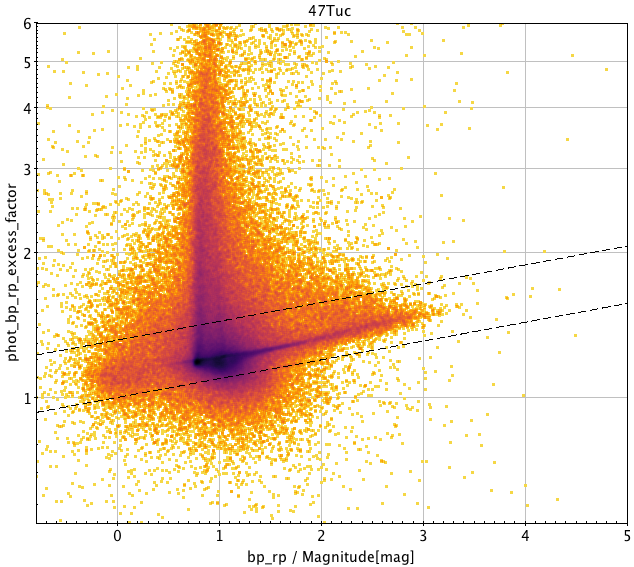8.2.3 Photometry

The construction of the $G$, $G_{\rm BP}$ and $G_{\rm RP}$ photometry is described in Riello et al. (2020), which also discusses the data quality and various issues detected. In addition, the general Gaia EDR3 validation paper (Fabricius et al. 2021) describes other important properties of the photometry, in particular its failure in certain cases. In one issue, this produced flawed photometry such that it was necessary that the photometry for more than 5 million sources in Gaia EDR3 was eliminated. For sources without $G$ photometry alternative photometry is available in a separate table published in the Gaia EDR3 known issues page.

The photometric uncertainties quoted in the catalogue are derived from the scatter of the calibrated fluxes. When only few observations are available, the quoted uncertainties themselves become uncertain since the uncertainties on the uncertainty estimates become larger. For $G_{\rm BP}$ and also for $G_{\rm RP}$ there are three cases where the derived uncertainty is zero. This has the side effect, that phot_bp_mean_flux_over_error or phot_rp_mean_flux_over_error become undefined (null).

An important point is that $G_{\rm BP}$ and $G_{\rm RP}$ photometry in Gaia EDR3 is a simple aperture photometry of prism spectra, assuming the source to be an isolated point source. Blending issues therefore arise for binaries as well as for sources in crowded regions. The specific issues for the observed colours are also discussed in Section 5.4.2. As it is shown in Fabricius et al. (2021), the excess factors have improved with respect to Gaia DR2. We show in Figure 8.6 some complementary plots showing the phot_bp_rp_excess_factor parameter computed as the flux ratio $C=(I_{BP}+I_{RP})/I_{G}$, like in Gaia DR2. The locus of well behaved stars is approximately given by log${}_{\rm 10}C=0.05+0.039(G_{\rm BP}-G_{\rm BP})$. Riello et al. (2020) define the locus of the well behaved sources through a polynomial and a corrected excess factor by substracting such polynomial. DPAC has not provided a general recommendation for a threshold on BP/RP flux excess and every kind of objects and/or stellar population and/or sky region may need a specific study to derive the most convenient selection according to the user interests.Figure 8.6: The excess flux phot_bp_rp_excess_factor computed from mean photometry for different subsamples of the catalogue. The dashed lines indicate the limits given by logC10=0.001+0.039⁢(GBP-GRP) and logC10=0.12+0.039⁢(GBP-GRP), see Riello et al. (2020)

There are 54,125 sources with no $G$ photometry. The cause is the low number of valid transits for the calculation of the mean photometry.

The results of first validations showed the presence of unrealistically faint sources, down to $G$ $\sim 25.6$ mag. The root cause was traced to poor quality spectral shape coefficients (SSCs). From the $G_{\rm BP}$ spectrum four coefficients are derived: bp_ssc0,…, bp_ssc3, and four similar from RP. The problem is that quotients like bp_ssc0/(bp_ssc1+bp_ssc2) are used in the calibration model without taking into account that the denominator in certain cases of poor quality SSCs may become extremely small and the quotient therefore extremely large. This is illustrated in Figure 8.7, where we can see that in the majority of cases (in fact 82% for this sample of very faint sources) the principal blue and red quotients have reasonable values (lower left), and that these values are well separated from cases of very large values.Figure 8.7: Quotients of blue and red spectral shape coefficient for a set of sources fainter than G ∼21.7 mag.

Studies like this have led us to not publish

• $G$ and $G_{\rm BP}$ photometry if the quotient bp_ssc0/(bp_ssc1+bp_ssc2) exceeds 6 or the quotient bp_ssc3/(bp_ssc1+bp_ssc2 exceeds 100;

• $G$ and $G_{\rm RP}$ photometry if the quotient rp_ssc0/(rp_ssc1+rp_ssc2 exceeds 30 or the quotient rp_ssc3/(rp_ssc1+rp_ssc2) exceeds 50.

The effect of such a photometry filter affects some 5 million sources and it is illustrated in Figure 8.8. The observations of those sources have been reprocessed with default values of their SSCs (as bronze sources) and their mean $G$ flux and magnitude are published, as mentioned above, separately in the Gaia EDR3 known issues page.Figure 8.8: Comparison of the G magnitude to the on-board detection for faint sources before (left) and after (right) applying the proposed photometric filter.